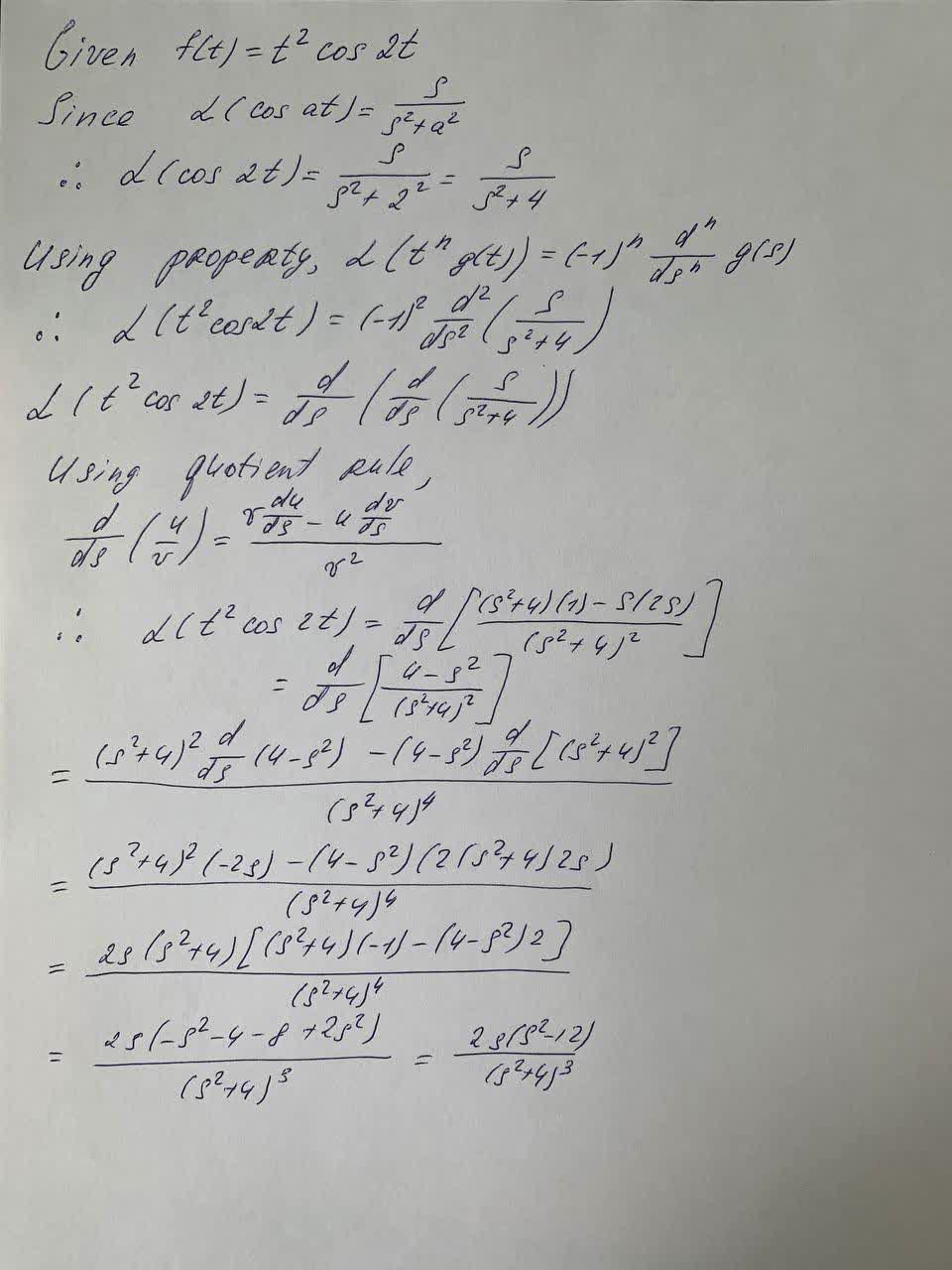find the Laplace transform of f (t). f(t)=t^2 \cos 2tremolatg 2021-09-23 Answered
find the Laplace transform of f (t).
$$\displaystyle{f{{\left({t}\right)}}}={t}^{{2}}{\cos{{2}}}{t}$$

Want to know more about Laplace transform?

• Questions are typically answered in as fast as 30 minutes

Solve your problem for the price of one coffee

• Math expert for every subject
• Pay only if we can solve itElberte